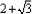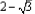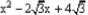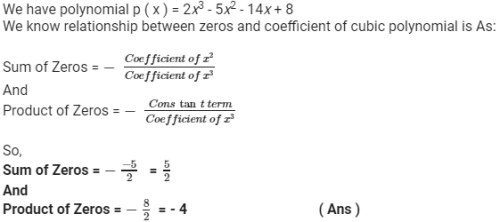Polynomials - MCQ Test

# Polynomials - MCQ Test

Test Description

## 10 Questions MCQ Test Quantitative Aptitude for Banking Preparation | Polynomials - MCQ Test

Polynomials - MCQ Test for Quant 2023 is part of Quantitative Aptitude for Banking Preparation preparation. The Polynomials - MCQ Test questions and answers have been prepared according to the Quant exam syllabus.The Polynomials - MCQ Test MCQs are made for Quant 2023 Exam. Find important definitions, questions, notes, meanings, examples, exercises, MCQs and online tests for Polynomials - MCQ Test below.
Solutions of Polynomials - MCQ Test questions in English are available as part of our Quantitative Aptitude for Banking Preparation for Quant & Polynomials - MCQ Test solutions in Hindi for Quantitative Aptitude for Banking Preparation course. Download more important topics, notes, lectures and mock test series for Quant Exam by signing up for free. Attempt Polynomials - MCQ Test | 10 questions in 20 minutes | Mock test for Quant preparation | Free important questions MCQ to study Quantitative Aptitude for Banking Preparation for Quant Exam | Download free PDF with solutions
 1 Crore+ students have signed up on EduRev. Have you?
Polynomials - MCQ Test - Question 1

### If the quotient and remainder were 3y - 5 and 9y + 10, on dividing 3y3 + y2 + 2y + 5 by g(y). Find g(y)

Detailed Solution for Polynomials - MCQ Test - Question 1 3Y3+Y2+2Y+5=(aY2+bY+c)(3Y-5)+9Y+19
=3aY3+(3b-5a)Y2+(3c-3b+9)Y-5c+10

Equate coefficient of Y3, Y2, Y, we get
a=1, b=2, and c=1
Hence,
aY2+bY+c=Y2+2Y+1
Polynomials - MCQ Test - Question 2

### Obtain the zeroes of the quadratic polynomial pqx2 + (q2 - Pr)x - qr

Polynomials - MCQ Test - Question 3

### If two of the zeroes areand. Find the other zeroes of t4 - 6t3 - 26t2 + 138t - 35

Polynomials - MCQ Test - Question 4

If p and q are the zeroes of the quadratic polynomial 4x2 - 1 = 0. Find the value of p2 + q2

Polynomials - MCQ Test - Question 5

Find the zeroes of the quadratic polynomial x2 + 19 x + 90

Polynomials - MCQ Test - Question 6

Find the sum and product of zeroes of the polynomialPolynomials - MCQ Test - Question 7

Find the zeroes of the polynomial x2 - 21

Polynomials - MCQ Test - Question 8

Find the sum and product of the zeroes of polynomial x2 - 51

Polynomials - MCQ Test - Question 9

If p and q are the zeroes of the polynomial x2 - 5x - k. Such that p - q = 1, find the value of K

Polynomials - MCQ Test - Question 10

Find the sum and product of the zeroes of cubic polynomial 2x3 - 5x2 - 14x + 8.

Detailed Solution for Polynomials - MCQ Test - Question 10## Quantitative Aptitude for Banking Preparation

66 videos|50 docs|90 tests
 Use Code STAYHOME200 and get INR 200 additional OFF Use Coupon Code
Information about Polynomials - MCQ Test Page
In this test you can find the Exam questions for Polynomials - MCQ Test solved & explained in the simplest way possible. Besides giving Questions and answers for Polynomials - MCQ Test, EduRev gives you an ample number of Online tests for practice

## Quantitative Aptitude for Banking Preparation

66 videos|50 docs|90 tests